EXAMPLE 1 Find a common monomial factor Factor

• Slides: 15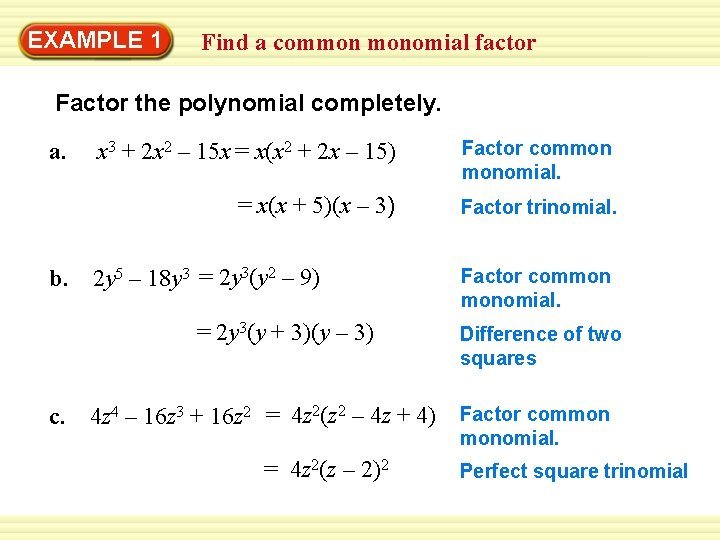EXAMPLE 1 Find a common monomial factor Factor the polynomial completely. a. b. x 3 + 2 x 2 – 15 x = x(x 2 + 2 x – 15) Factor common monomial. = x(x + 5)(x – 3) Factor trinomial. 2 y 5 – 18 y 3 = 2 y 3(y 2 – 9) = 2 y 3(y + 3)(y – 3) c. Factor common monomial. Difference of two squares 4 z 4 – 16 z 3 + 16 z 2 = 4 z 2(z 2 – 4 z + 4) Factor common monomial. = 4 z 2(z – 2)2 Perfect square trinomial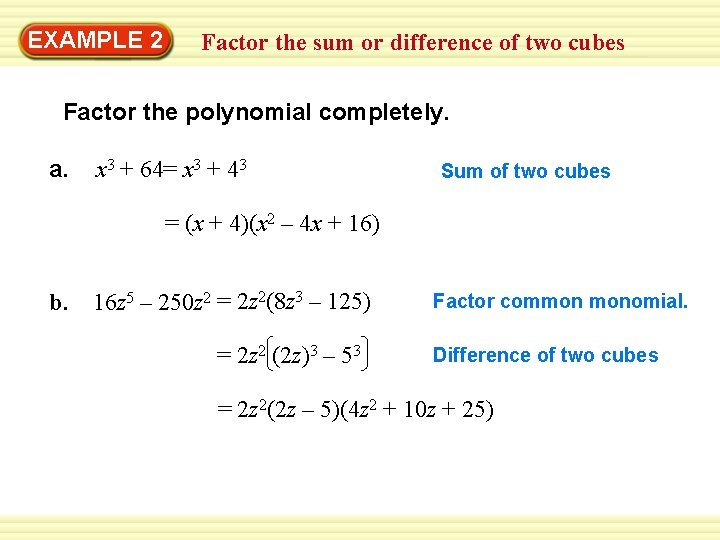EXAMPLE 2 Factor the sum or difference of two cubes Factor the polynomial completely. a. x 3 + 64= x 3 + 43 Sum of two cubes = (x + 4)(x 2 – 4 x + 16) b. 16 z 5 – 250 z 2 = 2 z 2(8 z 3 – 125) = 2 z 2 (2 z)3 – 53 Factor common monomial. Difference of two cubes = 2 z 2(2 z – 5)(4 z 2 + 10 z + 25)EXAMPLE 3 Factor by grouping Factor the polynomial x 3 – 3 x 2 – 16 x + 48 completely. x 3 – 3 x 2 – 16 x + 48 = x 2(x – 3) – 16(x – 3) Factor by grouping. = (x 2 – 16)(x – 3) Distributive property = (x + 4)(x – 3) Difference of two squaresEXAMPLE 4 Factor polynomials in quadratic form Factor completely: (a) 16 x 4 – 81 and (b) 2 p 8 + 10 p 5 + 12 p 2. a. 16 x 4 – 81 = (4 x 2)2 – 92 Write as difference of two squares. = (4 x 2 + 9)(4 x 2 – 9) Difference of two squares = (4 x 2 + 9)(2 x + 3)(2 x – 3) Difference of two squares b. 2 p 8 + 10 p 5 + 12 p 2 = 2 p 2(p 6 + 5 p 3 + 6) = 2 p 2(p 3 + 3)(p 3 + 2) Factor common monomial. Factor trinomial in quadratic form.EXAMPLE 5 Standardized Test Practice SOLUTION 3 x 5 + 15 x = 18 x 3 3 x 5 – 18 x 3 + 15 x = 0 3 x(x 4 – 6 x 2 + 5) = 0 Write original equation. Write in standard form. Factor common monomial.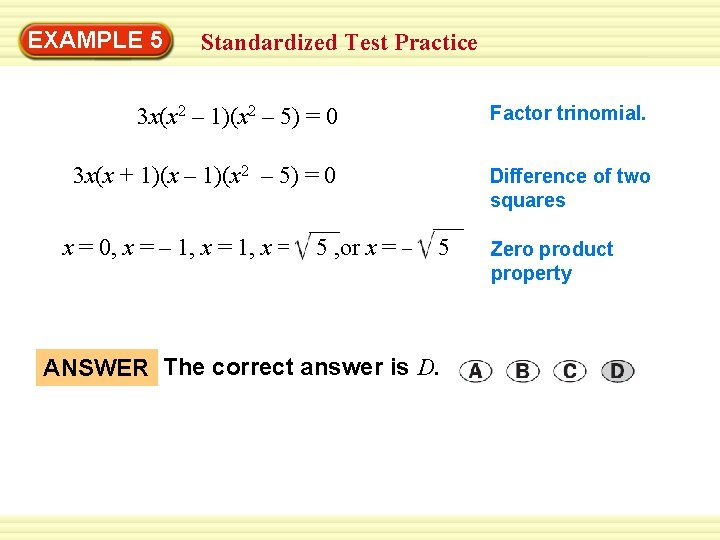EXAMPLE 5 Standardized Test Practice 3 x(x 2 – 1)(x 2 – 5) = 0 Factor trinomial. 3 x(x + 1)(x – 1)(x 2 – 5) = 0 Difference of two squares x = 0, x = – 1, x = 5 , or x = – 5 ANSWER The correct answer is D. Zero product propertyEXAMPLE 1 Use polynomial long division Divide f (x) = 3 x 4 – 5 x 3 + 4 x – 6 by x 2 – 3 x + 5. SOLUTION Write polynomial division in the same format you use when dividing numbers. Include a “ 0” as the coefficient of x 2 in the dividend. At each stage, divide the term with the highest power in what is left of the dividend by the first term of the divisor. This gives the next term of the quotient.EXAMPLE 1 Use polynomial long division 3 x 2 + 4 x – 3 x 2 – 3 x + 5 )3 x 4 – 5 x 3 + 0 x 2 + 4 x – 6 3 x 4 – 9 x 3 + 15 x 2 quotient Multiply divisor by 3 x 4/x 2 = 3 x 2 4 x 3 – 15 x 2 + 4 x Subtract. Bring down next term. 4 x 3 – 12 x 2 + 20 x Multiply divisor by 4 x 3/x 2 = 4 x – 3 x 2 – 16 x – 6 – 3 x 2 + 9 x – 15 – 25 x + 9 Subtract. Bring down next term. Multiply divisor by – 3 x 2/x 2 = – 3 remainderEXAMPLE 1 ANSWER Use polynomial long division 3 x 4 – 5 x 3 + 4 x – 6 = 3 x 2 + 4 x – 3 + – 25 x + 9 x 2 – 3 x + 5 CHECK You can check the result of a division problem by multiplying the quotient by the divisor and adding the remainder. The result should be the dividend. (3 x 2 + 4 x – 3)(x 2 – 3 x + 5) + (– 25 x + 9) = 3 x 2(x 2 – 3 x + 5) + 4 x(x 2 – 3 x + 5) – 3(x 2 – 3 x + 5) – 25 x + 9 = 3 x 4 – 9 x 3 + 15 x 2 + 4 x 3 – 12 x 2 + 20 x – 3 x 2 + 9 x – 15 – 25 x + 9 = 3 x 4 – 5 x 3 + 4 x – 6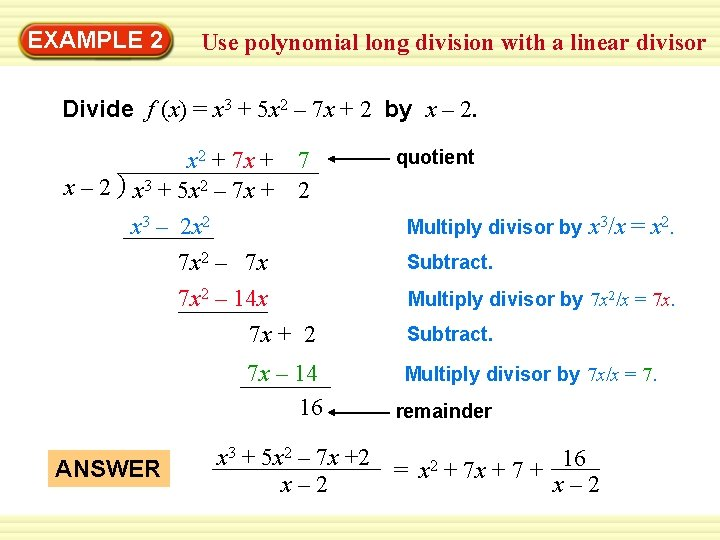EXAMPLE 2 Use polynomial long division with a linear divisor Divide f (x) = x 3 + 5 x 2 – 7 x + 2 by x – 2. x 2 + 7 x + 7 x – 2 ) x 3 + 5 x 2 – 7 x + 2 x 3 – 2 x 2 7 x 2 – 7 x 7 x 2 – 14 x 7 x + 2 7 x – 14 16 ANSWER quotient Multiply divisor by x 3/x = x 2. Subtract. Multiply divisor by 7 x 2/x = 7 x. Subtract. Multiply divisor by 7 x/x = 7. remainder x 3 + 5 x 2 – 7 x +2 = x 2 + 7 x + 7 + 16 x– 2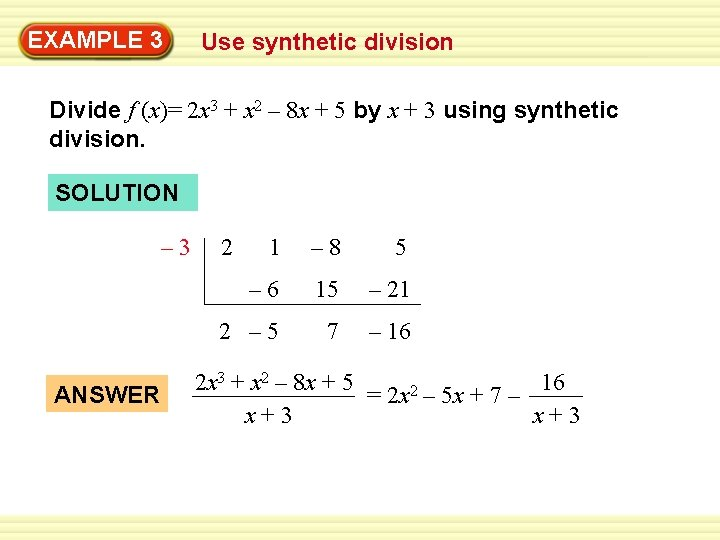EXAMPLE 3 Use synthetic division Divide f (x)= 2 x 3 + x 2 – 8 x + 5 by x + 3 using synthetic division. SOLUTION – 3 ANSWER 2 1 – 8 5 – 6 15 – 21 2 – 5 7 – 16 2 x 3 + x 2 – 8 x + 5 16 = 2 x 2 – 5 x + 7 – x+3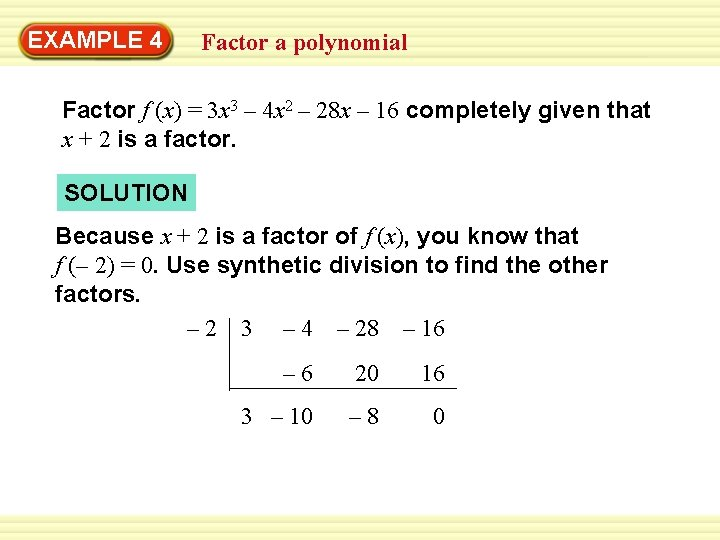EXAMPLE 4 Factor a polynomial Factor f (x) = 3 x 3 – 4 x 2 – 28 x – 16 completely given that x + 2 is a factor. SOLUTION Because x + 2 is a factor of f (x), you know that f (– 2) = 0. Use synthetic division to find the other factors. – 2 3 – 4 – 28 – 16 – 6 20 16 3 – 10 – 8 0EXAMPLE 4 Factor a polynomial Use the result to write f (x) as a product of two factors and then factor completely. f (x) = 3 x 3 – 4 x 2 – 28 x – 16 Write original polynomial. = (x + 2)(3 x 2 – 10 x – 8) Write as a product of two factors. = (x + 2)(3 x + 2)(x – 4) Factor trinomial.EXAMPLE 5 Standardized Test Practice SOLUTION Because f (3) = 0, x – 3 is a factor of f (x). Use synthetic division. 3 1 – 23 3 1 60 3 – 60 1 – 20 0EXAMPLE 5 Standardized Test Practice Use the result to write f (x) as a product of two factors. Then factor completely. f (x) = x 3 – 2 x 2 – 23 x + 60 = (x – 3)(x 2 + x – 20) = (x – 3)(x + 5)(x – 4) The zeros are 3, – 5, and 4. ANSWER The correct answer is A.